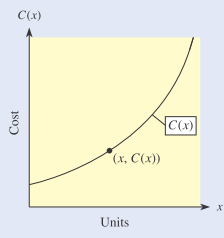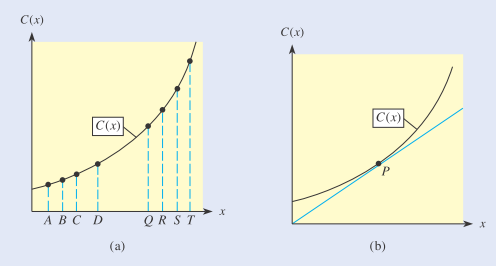# III. Tangent Lines and Optimization in Business and Economics In business and economics, common questions of interest include how to maximize profit or revenue, how to minimize average costs, and how to maximize average productivity. For questions such as these, the description of how to obtain the desired maximum or minimum represents an optimal (or best possible) solution to the problem. And the process of finding an optimal solution is called optimization. Answering these questions often involves tangent lines. In this project, we examine how tangent lines can be used to minimize average costs. Suppose that Wittage, Inc., manufactures paper shredders for home and office use and that its weekly total costs (in dollars) for x shredders are given by C ( x ) = 0.03 x 2 + 12.75 x + 6075 The average cost per unit for x units [denoted by C ¯ ( x ) ] is the total cost divided by the number of units. Thus, the average cost function for Wittage, Inc., is C ¯ ( x ) = C ( x ) x = 0.03 x 2 + 12.75 x + 6075 x = 0.03 x 2 x + 12.75 x x + 6075 x C ¯ ( x ) = 0.03 x + 12.75 + 6075 x Wittage, Inc., would like to know how the company can use marginal costs to gain information about minimizing average costs. To investigate this relationship, answer the following questions. Next, let’s examine graphically the relationship that emerged in Question 7 to see whether it is true in general. Figure 9.41 shows a typical total cost function (Wittage’s total cost function has a similar shape) and an arbitrary point on the graph. Figure 9.41 Figure 9.42 Figure 9.42(a) shows a typical total cost function with several points labeled. Explain how you can tell at a glance that C ¯ ( x ) is decreasing as the level of production moves from A to B to C to D . Explain how you can tell at a glance that C ¯ ( x ) is increasing as the level of production moves from Q to R to S to T .### Mathematical Applications for the ...

11th Edition
Ronald J. Harshbarger + 1 other
Publisher: Cengage Learning
ISBN: 9781305108042

#### Solutions

Chapter
Section### Mathematical Applications for the ...

11th Edition
Ronald J. Harshbarger + 1 other
Publisher: Cengage Learning
ISBN: 9781305108042
Chapter 9, Problem 9EAGP3
Textbook Problem
1 views

## III. Tangent Lines and Optimization in Business and EconomicsIn business and economics, common questions of interest include how to maximize profit or revenue, how to minimize average costs, and how to maximize average productivity. For questions such as these, the description of how to obtain the desired maximum or minimum represents an optimal (or best possible) solution to the problem. And the process of finding an optimal solution is called optimization. Answering these questions often involves tangent lines. In this project, we examine how tangent lines can be used to minimize average costs.Suppose that Wittage, Inc., manufactures paper shredders for home and office use and that its weekly total costs (in dollars) for x shredders are given by C ( x ) = 0.03 x 2 + 12.75 x + 6075 The average cost per unit for x units [denoted by C ¯ ( x ) ] is the total cost divided by the number of units. Thus, the average cost function for Wittage, Inc., is C ¯ ( x ) = C ( x ) x = 0.03 x 2 + 12.75 x + 6075 x = 0.03 x 2 x + 12.75 x x + 6075 x C ¯ ( x ) = 0.03 x + 12.75 + 6075 x Wittage, Inc., would like to know how the company can use marginal costs to gain information about minimizing average costs. To investigate this relationship, answer the following questions.Next, let’s examine graphically the relationship that emerged in Question 7 to see whether it is true in general. Figure 9.41 shows a typical total cost function (Wittage’s total cost function has a similar shape) and an arbitrary point on the graph.Figure 9.41Figure 9.42Figure 9.42(a) shows a typical total cost function with several points labeled. Explain how you can tell at a glance that C ¯ ( x ) is decreasing as the level of production moves from A to B to C to D. Explain how you can tell at a glance that C ¯ ( x ) is increasing as the level of production moves from Q to R to S to T.

This textbook solution is under construction.

### Still sussing out bartleby?

Check out a sample textbook solution.

See a sample solution

#### The Solution to Your Study Problems

Bartleby provides explanations to thousands of textbook problems written by our experts, many with advanced degrees!

Get Started